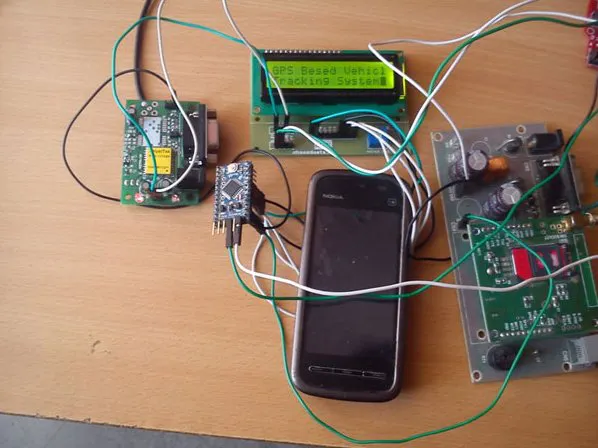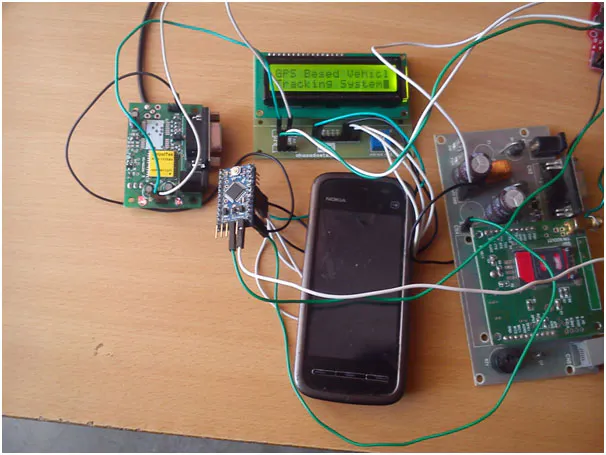# GPS and GSM-Based Vehicle Tracking System Using Arduino

This vehicle tracking system uses a GSM module to send the coordinates of vehicle to a mobile phone via text message.

IntermediateFull instructions provided9,961## Schematics

### Source Code of GPS and GSM based Vehicle Tracking System Using Arduino## Code

### GPS and GSM based Vehicle Tracking System Using Arduino

C/C++
```#include<LiquidCrystal.h>
LiquidCrystal lcd(12, 11, 5, 4, 3, 2);
char str;
char *test="\$GPGGA";
char logitude;
char latitude;
int i,j,k;
int temp;
int Ctrl+z=26;    //for sending msg
void setup()
{
lcd.begin(16,2);
Serial.begin(9600);
lcd.setCursor(0,0);
lcd.print("GPS Besed Vehicle ");
lcd.setCursor(0,1);
lcd.print("Tracking System");
delay(10000);
}
void loop()
{
if (temp==1)
{
for(i=18;i<27;i++)          //extract latitude from string
{
latitude[j]=str[i];
j++;
}
for(i=30;i<40;i++)          //extract longitude from string
{
logitude[k]=str[i];
k++;
}
lcd.setCursor(0,0);        //display latitude and longitude on 16X2 lcd display
lcd.print("Lat(N)");
lcd.print(latitude);
lcd.setCursor(0,1);
lcd.print("Lon(E)");
lcd.print(logitude);
delay(100);
Serial.println("AT+CMGF=1");    //select text mode
delay(10);
Serial.println("AT+CMGS=\"9610126059\"");  // enter receipent number
Serial.println();
Serial.print("Latitude(N): ");             //enter latitude in msg
Serial.println(latitude);                  //enter latitude value in msg
Serial.print("Longitude(E): ");            //enter Longitude in Msg
Serial.println(logitude);                  //enter longitude value in msg
Serial.write(Ctrl+z);                      //send msg  Ctrl+z=26
temp=0;
i=0;
j=0;
k=0;
delay(20000);                        // next reading within 20 seconds
}
}
void serialEvent()
{
while (Serial.available())            //Serial incomming data from GPS
{
str[i]= inChar;                  //store incomming data from GPS to temparary string str[]
i++;
if (i < 7)
{
if(str[i-1] != test[i-1])         //check for right string
{
i=0;
}
}
if(i >=60)
{
temp=1;
}
}
}
```

## Credits

### TechnicalEngineer

4 projects • 29 followers